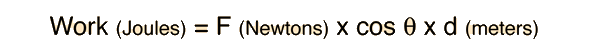# Work and the Work-Energy PrincipleEnter data in the boxes below. When you click outside the data box, the calculation will be made.

Start with a mass m of kg and an initial velocity v0= m/s.
Force angle = degrees.Work = J= N x x m.Final kinetic energy = J = Initial KEJ + Net workJ
From the final kinetic energy, the final velocity is v = m/s.
Index

Work-energy principle

 HyperPhysics***** Mechanics R Nave
Go Back